##### Basic Concepts

The wind is one of the most important actions to be taken into account in a lightweight tensile structure. Except those which are placed in interior in a decorative way, we always consider the action of wind.

In some areas of the World, this action is of vital importance, while in others it can go to second order behind the action of snow.

Although there is no specific wind regulations for tensile structures in most countries, if there are other regulations or basic criteria that we have to meet in order to quantify this action:

1. The force produced by the action of the wind is proportional to the square of the wind speed. For example, in a zone, wind speed of 100 km/h produces a suction of 40 kg/m². In the same area, wind from the same direction and speed 140 km/h will produce a suction of 80 kg/m². To determine for the wind speed we consider the regulations (if any) or the data supplied by the weather stations. Obtained the wind speed varies, or is affected by:
• the height above the ground: the higher elevation from the ground, the higher wind speed.
• the environment: the smoother, the higher wind speed.
• The form of tensile structure: creation of turbulence. We need physical tests to take this into account,  such as for example submit a mockup to a wind tunnel.
2. The force produced by the action of the wind is perpendicular to the surface of the membrane. Taking into account that the membrane is almost never flat, we will have to calculate the normal (perpendicular) vector to the membrane at the point where you want to apply the force of the wind. Considering that the membrane in Wintess3 is represented by the Elements, then we must look for the normal to each element.
3. The force produced by the action of the wind is a function of the angle formed by the normal vector to the surface (the element) and the vector that represents wind speed. A coefficient, Cp, called wind coefficient is determined using this angle.

One last but important remark. To apply the action of the wind it is necessary that the membrane exists. Otherwise WinTess3 considers the structure as a mesh of cables and only supports loads of self weight or applied directly to nodes.

##### WinTess3 and wind

Applying an action of wind in the WinTess3 program can be done in different ways. All of them are defined through the window of surface loads which can be accessed by using the F6 key or the menu: Calculate | Surface loads.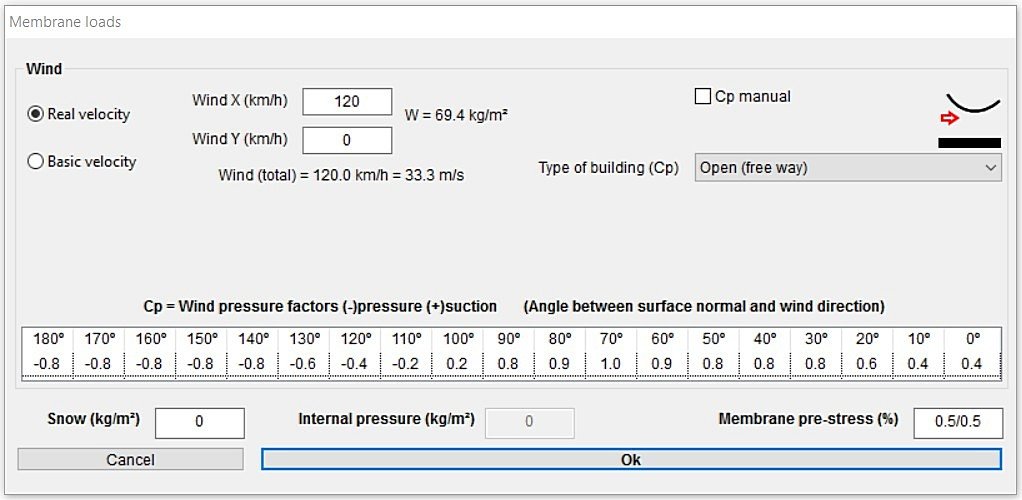First we have to enter the speed and direction of the wind. The wind is horizontal and therefore only has components X, Y. These components can be positive or negative, as they correspond to the positive or negative direction of the axes X and Y.

For example, a wind defined with X = 120 and Y = – 140 (assuming X and Y are eastward and northward, respectively) is a wind direction approximately South-East and with a speed of 184 km/h = √ (120² + 140²) km/h.

WinTess3 supports two types of wind speeds:

1. Real velocity
It is the maximum speed that, we assume, will occur in the exact place where the tensile structure is installed. WinTess will use it to calculate the loads, therefore the accuracy of the calculation depends on its accuracy. This value can be obtained from the local meteorological service, but we will have to adapt it to the exact position of the building: up on a terrace, tucked away in a courtyard, etc.
2. Basic velocity
This is a statistical value. It is defined by the regulations in force in each country (which used to be accompanied by zoned maps) and usually corresponds to the characteristic value of the average wind speed over a period of 10 minutes.
If there are no regulations, nor the value of the basic velocity of the wind is recorded, it is recommended that you use the value of real speed.

If we choose the second option: basic velocity, the program slightly modifies the surface loads window: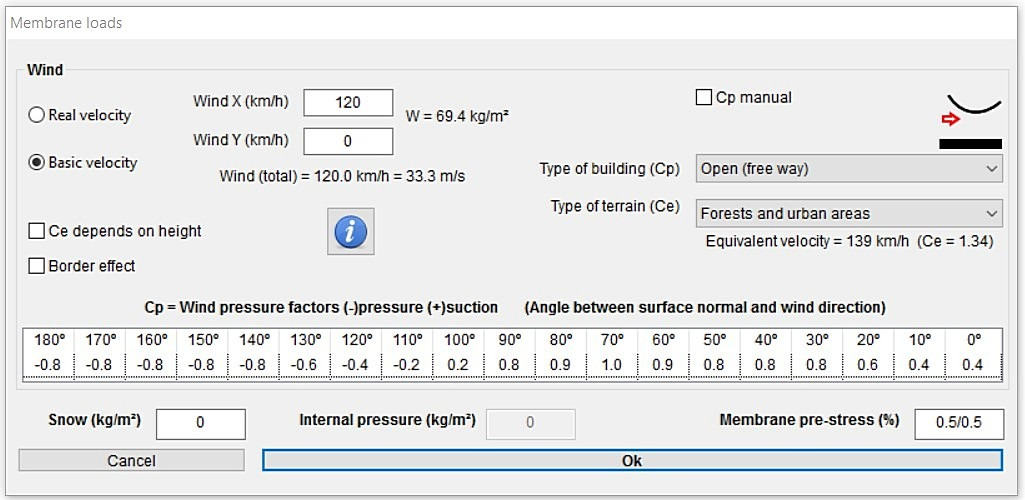New controls appear in the window:

• Ce depending on the height
Normally the regulation says us that the load produced by the wind increases with the height above the soil level. WinTess3 knows the height of every element, therefore it can apply this correction.
• Edge effect
It is known that perimeter edges, ridges or valleys of the membranes generate the effect of turbulence that increases the normal values of wind loads. The standards define what this increase should be and where it should be applied. WinTess3 simplifies this increase as follows:
Wind loads at all the elements of edge, ridge or valley are increased by 50 %. This is an empirical approach that facilitates the implementation of this concept.
If the mesh is very dense and the elements are very small, then the impact of edge effects will be unimportant. If the mesh is very large it will be opposite.
In any case, if we are not convinced by this approach, we can always modify the Cp for each element manually.
• Terrain type
The actual wind speed varies depending on the roughness of the terrain. The majority of standards define degrees of roughness in order to increase or reduce the calculated wind speed. Wintess3 considers following types of terrain:
• Sea or coastal area
• Flat area without obstacles
• Low vegetation and isolated obstacles
• Forests and urban area
• Among high buildings
• Help button
This button reminds us the following information: 1. The ground elevation must be Z = 0,  2. The basic “wind speed” is defined by the regulations (not the maximum!).

From this moment we can use one of two methods:

 1. Cp manual It is the typical method that is used when you have results of wind tunnel or a set of regulations that gives directly these values. In this case we mark the box of Cp manual and close the window of surface loads. Then we select menu Calculate | Cp manual (wind). The program will prompt you to pick a set of elements and apply a certain value of Cp. Normally, we will select items through a window defined by the mouse, but we can also select one by one. Once selected, the right mouse button will open the window that we see on the right. We can enter negative values for pressure or positive for suction. We must note well on this criterion because we can easily find the opposite criterion in other literature. IMPORTANT NOTE: You can also select Automatic Cp (see next paragraph) and then choose Manual Cp and change the value of Cp only for the elements that we want, leaving the rest same.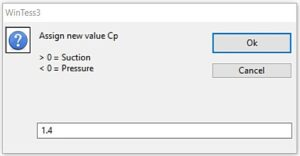2. Cp automatic In this case, we are going to use any of type of building the program provides . It must be kept in mind that what the program provides are proposals. The user must choose the one that best suits your approach or to the regulations (if any) in your country. It is advisable to use more than one type of building and opt for the most unfavourable. The user is responsible for the wind coefficient values Cp that the program is going to use. For each type of building, the program proposes a series of coefficients wind Cp. The table itself tells us that these values are a function of the angle formed by the normal to the surface and the wind direction. Thus, in a building of vertical facades, in the rear facade, to leeward, match the direction and the wind direction and the normal. In this case, the angle is 0º. On the contrary, in the facade to windward, the direction is opposite, therefore the angle is 180º. All other cases will be provided between these values. Side vertical facades or a flat roof will have an angle of 90º.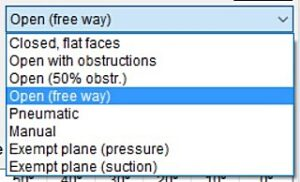(Manual)

If we are not satisfied by the values of Cp of any of the types of building that the program proposes, we can choose “Manual” type of building. In this case, and only in this case, the program allows you to modify 19 values of wind coefficient Cp manually.

Once the values of wind speed V and the Cp of the surface are defined, the program calculates the loads on the surface. It achieves this in the following manner:

Each element is a triangle whose plane has only one normal. The program automatically calculates the angle between this normal and the direction of the wind vector. Interpolating this value of angle with the 19 presets, we obtain the Cp value for the element (triangle).

If we have used the basic wind speed, we also have Ce value which depends on type of terrain and can be modified according to the actual element height, since both modify the speed of the wind. In the case in which the real wind speed, then Ce = 1.

Each element is a triangle with a given surface area S. The program divides this area into three parts S1, S2, S3 and assigns them to each of the vertices. This distribution is not equitable, but it resembles a distribution type Voronoi diagram, using the properties of the triangle.

A force in the direction of normal to the triangle is applied to each vertex, and module Fi = Si· Cp · Ce, being the part of surface of the element that corresponds to the vertex, or point in question with the Cp and Ce coefficients mentioned before. Doing this for all elements, each node will have an applied force of Fv = Σ F1 + F+…+ Fn, obtained by adding the node forces vectorially caused by all the triangles that share the same vertex in question. We can see the forces produced by the wind on the surface of the membrane through the button on the right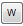.

##### Characteristic value

The characteristic value of wind speed, mentioned above, is defined as the value of which annual probability of being exceeded is 0.02 (return period of 50 years).
We can modify this value depending on the period of service with which the building is projected.

Correction of the basic rate depending on the period of service:

 Return period (years) 1 2 5 10 20 50 200 Correction factor 0,41 0,78 0,85 0,90 0,95 1,00 1,08